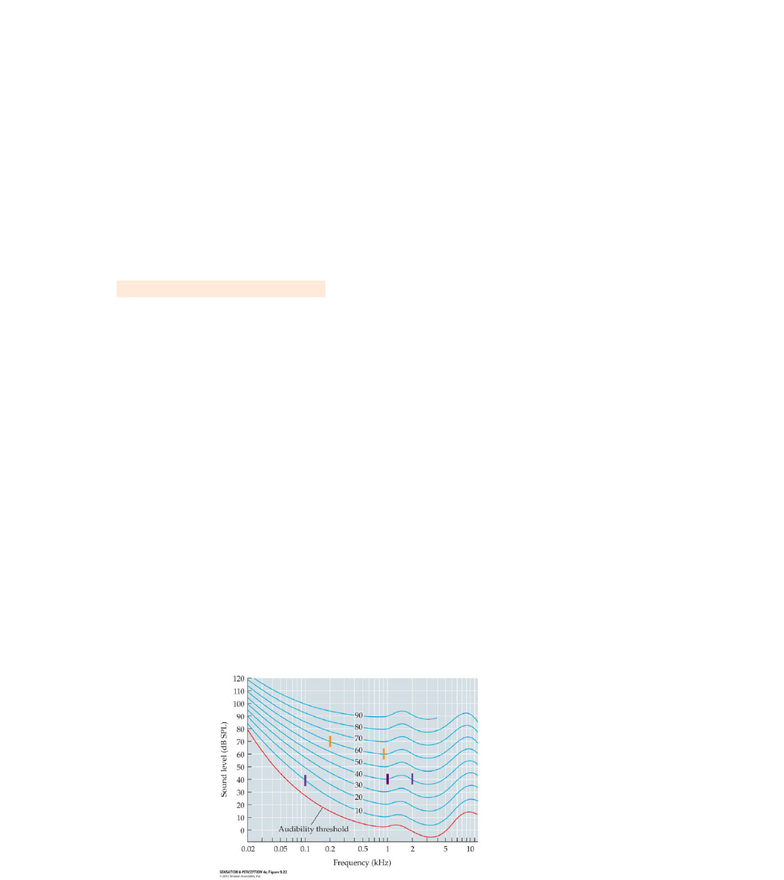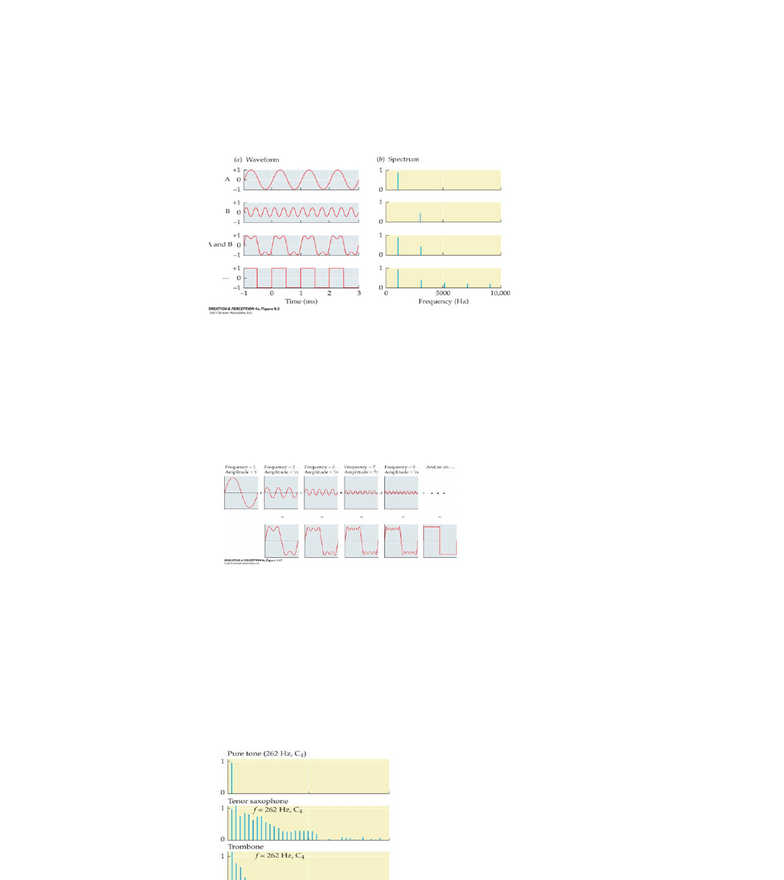# PSYC 212 Lecture Notes - Lecture 14: Sound Pressure, Phon, Logarithmic Scale

37 views6 pages

For unlimited access to Class Notes, a Class+ subscription is required.Decibels(and(loudness
The\$main\$determinant\$is\$the\$amplitude\$of\$waveform
loudness\$is\$generally\$measured\$in\$decibels
Formula:\$dB\$=\$20log(p/p0)
They\$are\$always\$relative\$to\$the\$smallest\$pressure\$perceivable
dB\$are
The\$reason\$why\$we\$need\$to\$square\$the\$pressure\$when\$calculating\$intensity:\$sound\$waves\$propagate\$in\$
3d.\$Intensity\$corresponds\$to\$the\$energy\$of\$the\$sound\$wave\$when\$it\$hits\$a\$2D\$surface,\$like\$the\$eardrum
MOST\$IMPORTANT\$TO\$REMEMBER:\$dB\$scale\$is\$relative\$to\$smallest\$pressure\$perceivable\$and\$it\$is\$a\$
logarithmic\$scale.
Frequency(and(pitch
PITCH=\$the\$psychological\$aspect\$of\$sound\$related\$mainly\$to\$the\$fundamental\$frequency\$
The\$frequency\$(Hz)\$=\$number\$of\$cycles\$per\$second
E.g.\$is\$there\$are\$2\$cycles\$in\$a\$second,\$the\$frequency\$is\$2Hz
Higher\$frequencies\$will\$be\$associated\$with\$high\$pitches,\$and\$vice\$versa.
Sounds\$that\$only\$have\$one\$frequency\$are\$called\$pure\$tones.
Audibility
Audibility\$of\$a\$sound\$is\$due\$to\$a\$mix\$of\$frequency\$and\$amplitude
AUDIBILITY=\$capacity\$to\$hear\$a\$sound
It's\$more\$difficult\$to\$hear\$a\$sound\$that\$has\$a\$low\$frequency
Music\$is\$in\$the\$middle\$of\$our\$audible\$range;\$you\$won't\$play\$music\$that\$can't\$be\$heard\$by\$someone
If\$sounds\$are\$too\$loud\$[amplitude\$is\$too\$high]\$the\$sound\$will\$become\$unpleasant\$and\$can\$even\$become\$
painful.
EQUAL-LOUDNESS\$CURVE=\$a\$graph\$plotting\$sound\$pressure\$level\$[dB\$SPL]\$against\$the\$frequency\$for\$
which\$a\$listener\$perceives\$constant\$loudness.
Note:\$the\$unit\$used\$is\$the\$'phon'\$which\$corresponds\$to.\$The.\$dB\$value\$of\$the\$curve\$at\$1k\$Hz
On\$the\$graph,\$decibels\$are\$calculated.\$Based\$on\$sound\$pressure:\$dB\$=\$20log(p/p0)\$
For\$instance,\$a\$sound\$of\$40dB\$is\$equal\$to\$40\$phons\$at\$1kHz\$and\$2kHz,\$but\$to\$10\$phons\$at\$0.1kHz
…\$or,\$sounds\$of\$70\$dB\$at\$0.2kHz\$and\$60dB\$at\$1kHz\$will\$sound\$equally\$lead\$[60\$phons]
§
Lecture\$14
Thursday, February 28, 2019
1:10 PM
Unlock document

This preview shows pages 1-2 of the document.
Unlock all 6 pages and 3 million more documents.

Already have an account? Log inFrequency
Complex\$sounds\$can\$be\$understood\$as\$a\$combination\$of\$several\$frequencies.\$In\$nature,\$every\$sound\$is\$a\$
complex\$sound.\$
Complex\$sounds\$can\$be\$described\$by\$their\$spectrum.\$
In\$the\$complex\$waveform,\$there\$is\$a\$lot\$of\$power\$at\$the\$lower\$frequency\$and\$a\$lot\$of\$power\$at\$the\$
other\$higher\$frequency.\$If\$you\$combine\$all\$the\$waveforms\$to\$the\$point\$where\$you\$have\$a\$square\$
wave:
§
The\$height\$of\$the\$bar\$=\$amplitude\$of\$the\$frequency
Location\$of\$bar\$on\$graph\$=\$the\$frequency\$we\$are\$characterizing
The\$first\$waveform\$is\$a\$pure\$tone;\$the\$second\$one\$is\$also\$a\$waveform;\$the\$third\$is\$adding\$
waveforms\$A\$and\$B,\$one\$bar\$corresponds\$to\$the\$first\$1k\$Hz\$and\$the\$second\$represents\$the\$other\$
frequency.\$\$So\$you\$can\$tell\$that\$there's\$a\$lot\$of\$one\$frequency\$and\$little\$of\$the\$other.\$The\$fourth\$
waveform:\$if\$you\$imagine\$that\$you\$add\$a\$higher\$and\$higher\$frequency\$with\$a\$lower\$amplitude,\$it's\$
going\$to\$lead\$to\$that.\$The\$more\$we\$add\$a\$frequency\$with\$a\$high\$intensity,\$the\$more\$the\$waveform\$
resembles\$a\$square:
§
Many\$sounds\$have\$a\$harmonic\$spectrum.
HARMONIC\$SPECTRUM=\$the\$spectrum\$of\$a\$complex\$sound\$in\$which\$energy\$is\$at\$integer\$multiples\$of\$the\$
fundamental\$frequency\$
Typically\$caused\$by\$a\$simple\$vibrating\$source\$[e.g.\$a\$string\$of\$a\$guitar,\$or\$reed\$of\$a\$saxophone]
FUNDAMENTAL\$FREQUENCY\$=\$the\$lowest-frequency\$component\$of\$a\$complex\$periodic\$sound
TIMBRE=\$the\$psychological\$sensation\$by\$which\$a\$listener\$can\$judge\$that\$two\$sounds\$with\$the\$
same\$loudness\$and\$pitch\$are\$dissimilar.
§
Timbre\$quality\$is\$conveyed\$by\$the\$profile\$of\$the\$harmonics.
For\$the\$four\$sounds\$here,\$the\$fundamental\$frequency\$is\$the\$same\$but\$the\$harmonics\$[overtone]\$
are\$what\$change:\$
§
Unlock document

This preview shows pages 1-2 of the document.
Unlock all 6 pages and 3 million more documents.

Already have an account? Log in

# Get access

Grade+
\$10 USD/m
Billed \$120 USD annually
Homework Help
Class Notes
Textbook Notes
40 Verified Answers
Study Guides
1 Booster Class
Class+
\$8 USD/m
Billed \$96 USD annually
Homework Help
Class Notes
Textbook Notes
30 Verified Answers
Study Guides
1 Booster Class